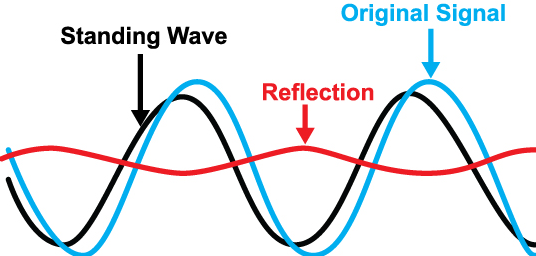# VSWR

Impedance mismatches in a radio-frequency (RF) electrical transmission line cause reflection of rf power and thus power loss. VSWR is a way to measure transmission line imperfections.

VSWR stands for Voltage Standing Wave Ratio, and is also referred to as Standing Wave Ratio (SWR). It is a measure of how efficiently RF power is transmitted from the power source, through the transmission line, and into the load. Maximum power transfer between two system components occurs when their respective impedances are matched. If the impedances are not identical, some RF power will be reflected back, resulting in a reduction in the amount of power delivered to the load. These reflections cause voltage standing waves. VSWR is defined as the ratio of the maximum voltage to the minimum voltage in the standing wave. The larger the impedance mismatch, the larger the amplitude of the standing wave.As seen from the above image, red color wave is the reflection from the load.

A common example is a BTS output port connected through a transmission line to an antenna. Suppose If there is no mismatch between the BTS output port and antenna port, then there will not be any reflections and thus VSWR will be equal to 1. And if there is mismatch between BTS output port and antenna port, then VSWR value will be greater than 1 depending upon the amount of mismatch.

Although the concept of VSWR is easy to comprehend, it is extremely difficult to measure directly. For that reason, you need to measure other parameters, such as return loss or reflection coefficient which can then be used to calculate VSWR.

Return loss (RL) is the difference in power (expressed in dB) between the incident power and the power reflected back by the load due to a mismatch.

Return loss (RL) = -10log(Preflected /Pincident )

Once the Return Loss is known, the next step is calculate reflection coefficient ( Γ ),

RL = -20 * Log (Γ )

Γ = 10 (-RL/20)

VSWR can then be calculated using the following formula:

VSWR = (1 + Γ ) / (1 – Γ)

Below is the VSWR table for reference:

 Return Loss (dB) Reflection Coeficient VSWR Return Loss (dB) Reflection Coeficient VSWR 49 0.0035 1.007 24 0.0631 1.135 48 0.0040 1.008 23 0.0708 1.152 47 0.0045 1.009 22 0.0794 1.173 46 0.0050 1.010 21 0.0891 1.196 45 0.0056 1.011 20 0.1000 1.222 44 0.0063 1.013 19 0.1122 1.253 43 0.0071 1.014 18 0.1259 1.288 42 0.0079 1.016 17 0.1413 1.329 41 0.0089 1.018 16 0.1585 1.377 40 0.0100 1.020 15 0.1778 1.433 39 0.0112 1.023 14 0.1995 1.499 38 0.0126 1.025 13 0.2239 1.577 37 0.0141 1.029 12 0.2512 1.671 36 0.0158 1.032 11 0.2818 1.785 35 0.0178 1.036 10 0.3162 1.925 34 0.0200 1.041 9 0.3548 2.100 33 0.0224 1.046 8 0.3981 2.323 32 0.0251 1.052 7 0.4467 2.615 31 0.0282 1.058 6 0.5012 3.010 30 0.0316 1.065 5 0.5623 3.570 29 0.0355 1.074 4 0.6310 4.419 28 0.0398 1.083 3 0.7079 5.848 27 0.0447 1.094 2 0.7943 8.724 26 0.0501 1.106 1 0.8913 17.391 25 0.0562 1.119 0 1.0000 infinity
Tagged